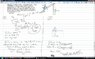# Yet another two-block system question. I guess I don't understand this still.

Bensky

## Homework Statement

See attempt at solution. It has everything.

## Homework Equations

F_net = ma (net force)
W = mg (weight)
f_k = u_k * N (frictional force - kinetic)

T is the tension force.

## The Attempt at a Solution

See attachment below. I realize I just posted a problem related to a two block system. I got help on the last problem and thought I understood it completely, but this time I'm REALLY not sure what I'm missing. I checked over the coordinate system and all the signs in the problem and thought all my steps made sense. Am I simply missing a force?

The answer I should be getting is u_k = 0.25, but I get 0.583 instead.

#### Attachments

•attempt2.jpg
56.5 KB · Views: 325
Last edited:

Homework Helper
For 10 kg block g*sinθ and ax are in the same direction. So in that equation you have to equate it to -m*ax.

Bensky
For 10 kg block g*sinθ and ax are in the same direction. So in that equation you have to equate it to -m*ax.

Thank you, that did give me the correct answer. Are you saying the acceleration should be negative on block A since it is traveling in the -x direction (left)?

Homework Helper
Thank you, that did give me the correct answer. Are you saying the acceleration should be negative on block A since it is traveling in the -x direction (left)?
The mass is moving in the direction of g*sinθ. In the equation you have taken g*sinθ -ve, so ax also -ve.

Bensky
The mass is moving in the direction of g*sinθ. In the equation you have taken g*sinθ -ve, so ax also -ve.

What is "ve"?

EDIT: Nevermind, you mean -ve = "negative" haha. I'm a little slow tonight, nevermind! I understand, thanks.